# T3 - page 15211

SVGuss:
Hi Guys,

I cannot code at all, but I managed to combine these two indicators I like (one made by fxbs, the other I don't know), so basically you have a T3MA that changes color, not when its angle changes (like in All_Averages_V2.2), but when it is penetrated by the price.

The RoundPrice indie is required for Ma_RoundPrice to work.

Enjoy.

Dear SVGuss

The indicator does not work.I tried to compile it on mt4 editor but I have an error message like this-"breakBars" variable non defined

Any idea how can be fixed??

Regards

Dan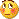186

dansmol:
Dear SVGuss

The indicator does not work.I tried to compile it on mt4 editor but I have an error message like this-"breakBars" variable non defined

Any idea how can be fixed??

Regards

DanHi dansmol,

Here is it fixed; You also need to have `RoundPriceNE_big_mod[5dig]` in the indicator folder. ( Sorry , I do not have the mq4 file)

Have a good WE

Tomcat

Files:186

mladen:
Boxter

I know what happened to the indicator from the post (it was deleted when I was "over pleased" one day with tro's beautiful work, and it was deleted by me) but now I can not find that version on my PC (it was long, long time ago ...)

Anyway, in the mean time, metatrader somehow managed to fix the bug they had with iStdDevOnArray() function so the original indicator can be used now since there is no need any more for a separate custom iStdDevOnArray() function

regards

Mladen

Hi,

That one?

KAMA in the indicator folder

PriceSeries in the include folder.

Hope it helps.

Have a good WE.

Tomcat

Files:
kama.mq4  7 kb216611

Tomcat

It is not the one (my had a custom deviations calculation that replaced the built in "on array" function) but thanks anyway.

As I said, the need for a custom deviation calculation does not exist any more since that error in metatrader was corrected in one of the updates, so the need for that version of Kaufman adaptive moving average indicator does not exist any more too

regards

Mladen

Tomcat98:
Hi,

That one?

KAMA in the indicator folder

PriceSeries in the include folder.

Hope it helps.

Have a good WE.

Tomcat211

Tomcat98:
Hi dansmol,

Here is it fixed; You also need to have `RoundPriceNE_big_mod[5dig]` in the indicator folder. ( Sorry , I do not have the mq4 file)

Have a good WE

Tomcat

THANK YOU VERY MUCH TOMCAT98

REGARDS

Dan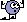133

Calculating t3_clean from the EA, and not the indicator

Hi every one ! I would like to be able to calculate different t3_clean value from the EA and not the indicator. so I can manipulate the last price used to calculate the indicator. I am using : t3_clean from mladen at https://www.mql5.com/en/forum/173058/page4.

Any help would be fanatastic.133

so in the t3_clean code we have this block of code :

double CalculateT3(int limit,int period,int priceType)

{

Print("This is the data in the T3"+"\t "+limit+"\t "+period+"\t "+priceType);

Print("Info Indicator from the Indicator "+IndicatorCounted() );

if (t3.period != period)

{

t3.period = period;

b2 = b*b;

b3 = b2*b;

c1 = -b3;

c2 = (3*(b2+b3));

c3 = -3*(2*b2+b+b3);

c4 = (1+3*b+b3+3*b2);

w1 = 2 / (2 + 0.5*(MathMax(1,period)-1));

w2 = 1 - w1;

}

//

//

//

//

//

for(int i=limit; i>=0; i--)

{

if(i == index_posi)

{

//v_manipul=

double price = v_manipul;

e1 = w1*price + w2*ae1;

e2 = w1*e1 + w2*ae2;

e3 = w1*e2 + w2*ae3;

e4 = w1*e3 + w2*ae4;

e5 = w1*e4 + w2*ae5;

e6 = w1*e5 + w2*ae6;

t3Array=c1*e6 + c2*e5 + c3*e4 + c4*e3;

ae1 = e1;

ae2 = e2;

ae3 = e3;

ae4 = e4;

ae5 = e5;

ae6 = e6;

}else{

price = iMA(NULL,0,1,0,MODE_SMA,priceType,i);

e1 = w1*price + w2*ae1;

e2 = w1*e1 + w2*ae2;

e3 = w1*e2 + w2*ae3;

e4 = w1*e3 + w2*ae4;

e5 = w1*e4 + w2*ae5;

e6 = w1*e5 + w2*ae6;

t3Array=c1*e6 + c2*e5 + c3*e4 + c4*e3;

ae1 = e1;

ae2 = e2;

ae3 = e3;

ae4 = e4;

ae5 = e5;

ae6 = e6;

}

}

}[/CODE]

I am trying to adapt it inside an Expert so it can be call to calculate any t3_clean value on demand, by changing the last bar value. e.g, the t3 for the bar 83.8167 is 85.9751; what if the bar was 81 and not 83 ? ect..., so so far, this is my code :

[CODE]

double CalculateT3(int limit,int period,int priceType,int index_posi, double v_manipul, int index_i)

{

double t3Array[];

double ae1[];

double ae2[];

double ae3[];

double ae4[];

double ae5[];

double ae6[];

ArrayResize( t3Array, limit);

ArrayResize( ae1, limit);

ArrayResize( ae2, limit);

ArrayResize( ae3, limit);

ArrayResize( ae4, limit);

ArrayResize( ae5, limit);

ArrayResize( ae6, limit);

Print("This is the data in the T3 FROM THE EA >>>>>> "+"\t "+limit+"\t "+period+"\t "+priceType);

Print("Info Indicator from the Indicator FROM THE EA <<<<<<<< "+IndicatorCounted() );

if (t3.period != period)

{

t3.period = period;

b2 = b*b;

b3 = b2*b;

c1 = -b3;

c2 = (3*(b2+b3));

c3 = -3*(2*b2+b+b3);

c4 = (1+3*b+b3+3*b2);

w1 = 2 / (2 + 0.5*(MathMax(1,period)-1));

w2 = 1 - w1;

}

Print("Voici w in the EA A VOIT XXXXXXXXX>>>XXXX<<>>"+w2+" "+w1);

//

//

//

//

//

for(int i=limit; i>=0; i--)

{

if(i == index_posi)

{

//v_manipul=

double price = v_manipul;

e1 = w1*price + w2*ae1;

e2 = w1*e1 + w2*ae2;

e3 = w1*e2 + w2*ae3;

e4 = w1*e3 + w2*ae4;

e5 = w1*e4 + w2*ae5;

e6 = w1*e5 + w2*ae6;

t3Array=c1*e6 + c2*e5 + c3*e4 + c4*e3;

ae1 = e1;

ae2 = e2;

ae3 = e3;

ae4 = e4;

ae5 = e5;

ae6 = e6;

Print("PREMIERE ETAPE DATA DANS LARRAY ]]]]]]]]]]]]]]}}}}} "+t3Array);

}else{

price = iMA(NULL,0,1,0,MODE_SMA,priceType,i);

e1 = w1*price + w2*ae1;

e2 = w1*e1 + w2*ae2;

e3 = w1*e2 + w2*ae3;

e4 = w1*e3 + w2*ae4;

e5 = w1*e4 + w2*ae5;

e6 = w1*e5 + w2*ae6;

t3Array=c1*e6 + c2*e5 + c3*e4 + c4*e3;

ae1 = e1;

ae2 = e2;

ae3 = e3;

ae4 = e4;

ae5 = e5;

ae6 = e6;

double op = c1*e6 + c2*e5 + c3*e4 + c4*e3;

Print("DEUXIEME ETAPE DATA DANS LARRAY ]]]]]]]]]]]]]]}}}}} "+op);

Print("SHOW ME PRICE "+ ae1);

}

}

return (t3Array);

}

and it is not working at all...anyone to help ?216611

T3 oscillator ...

First I though to make a version of awesome oscillator using T3, but then, when I tried it, it turned out that with awesome oscillator calculating lengths (5,14) it is too fast. So decided to open lengths as parameters and to use other default calculating lengths.

This is how it looks like with default parameters now :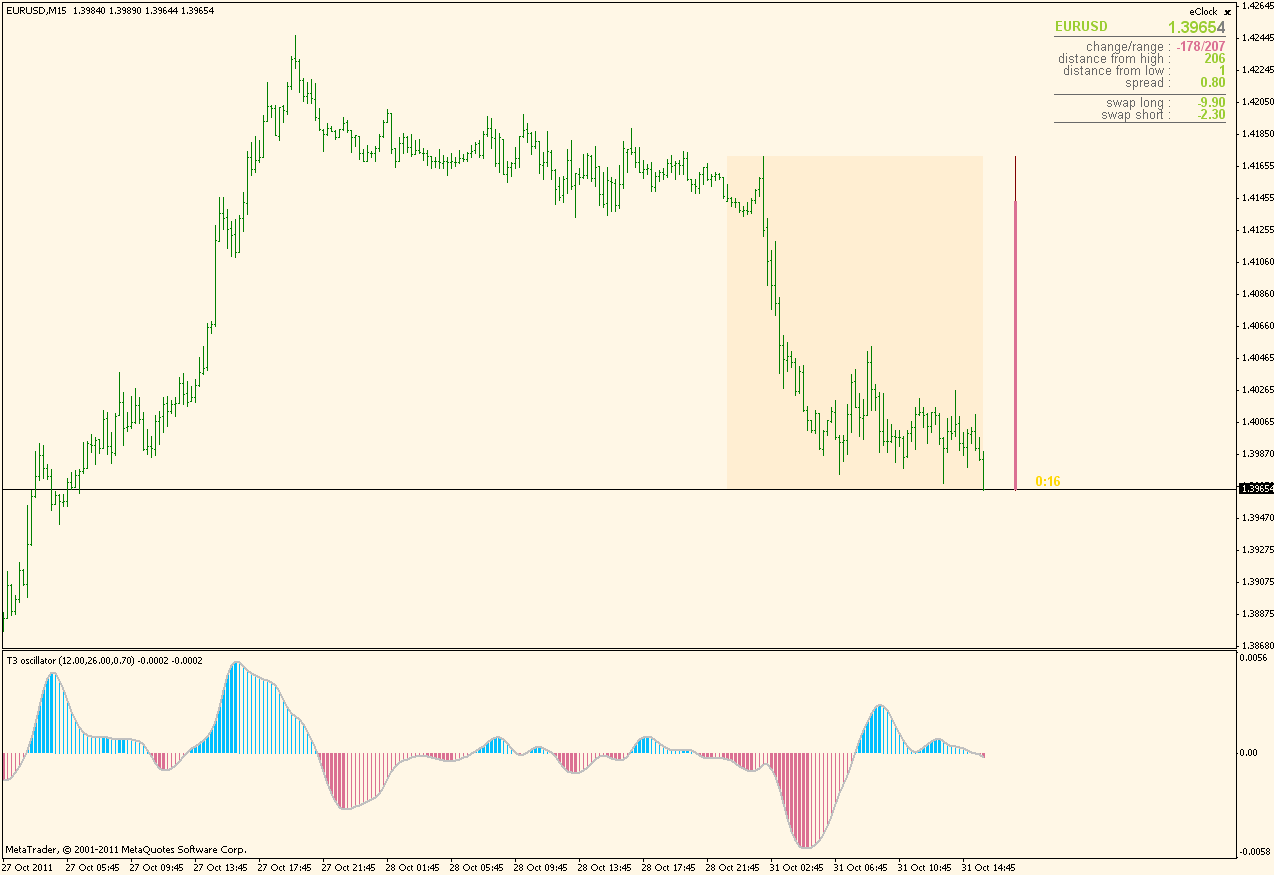Files: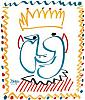1285

mladen:
First I though to make a version of awesome oscillator using T3, but then, when I tried it, it turned out that with awesome oscillator calculating lengths (5,14) it is too fast. So decided to open lengths as parameters and to use other default calculating lengths.

This is how it looks like with default parameters now :With fast/slow settings : 6/12 on a renko chart it's beautiful, could be a "simple" strategy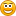Thanks mladen !216611

And one more T3 version : T3 GMMA

For short (shorter periods - faster) part set the ShowLongGmma parameter to false. For long (longer periods - slower) set it to true and the, by combining the 2 you can get something like this :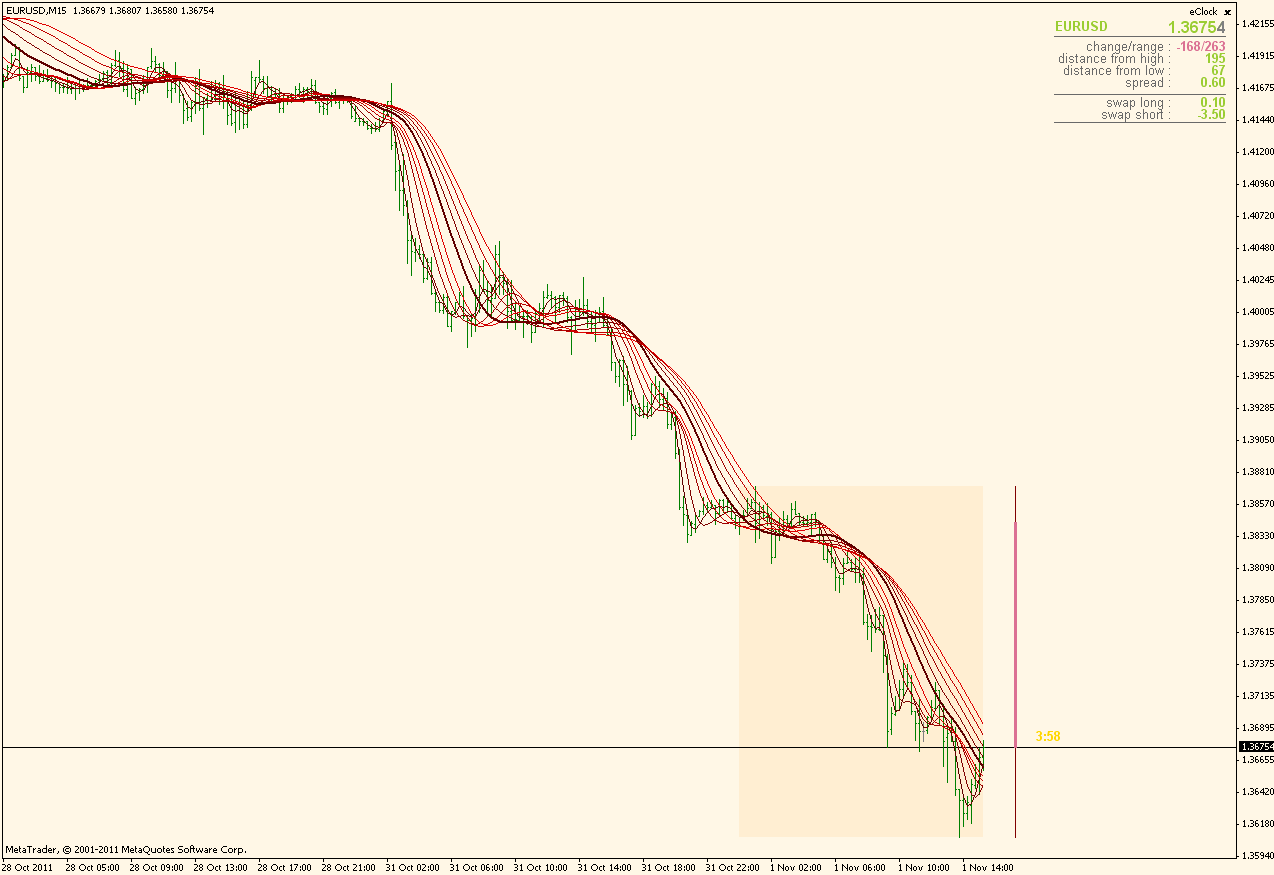Files:
t3_gmma.gif  29 kb
t3_gmma.mq4  5 kb